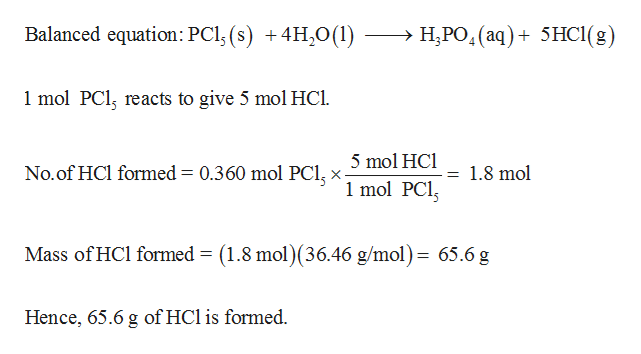# Be sure that all of the chemical equations are properly balanced.a.) Oxygen gas can be produced in the laboratory by decomposition of potassium chlorate (KClO3):KClO3 (s) → 2KCl (s) + 3O2 (g)How many kg of O2 can be produced from 1.0 kg of KClO3?b.) Phosphorus pentachloride reacts with water to give phosphoric acid and hydrogen chlorideaccording to the following unbalanced equation:PCl5 (s) + H2O(l) → H3PO4 (aq) + HCl (g)If 0.360 moles of PCl5 was slowly added to an excess amount of water, how many grams of HClwere formed? c.) An experiment was begun with 75.00 g of Ba(NO3)2 and 50.00 g of Na2SO4. After collecting anddrying the product, 64.45 g of BaSO4 was obtained. Calculate the theoretical yield andpercentage yield of BaSO4.Ba(NO3)2 (aq) + Na2SO4 (aq) → BaSO4 (s) + 2NaNO3 (aq)

Question
27 views

Be sure that all of the chemical equations are properly balanced.

a.) Oxygen gas can be produced in the laboratory by decomposition of potassium chlorate (KClO3):
KClO3 (s) → 2KCl (s) + 3O2 (g)
How many kg of O2 can be produced from 1.0 kg of KClO3?

b.) Phosphorus pentachloride reacts with water to give phosphoric acid and hydrogen chloride
according to the following unbalanced equation:
PCl5 (s) + H2O(l) → H3PO4 (aq) + HCl (g)
If 0.360 moles of PCl5 was slowly added to an excess amount of water, how many grams of HCl
were formed?

c.) An experiment was begun with 75.00 g of Ba(NO3)2 and 50.00 g of Na2SO4. After collecting and
drying the product, 64.45 g of BaSO4 was obtained. Calculate the theoretical yield and
percentage yield of BaSO4.
Ba(NO3)2 (aq) + Na2SO4 (aq) → BaSO4 (s) + 2NaNO3 (aq)

check_circle

Step 1

In a balanced equation the number of atoms of each element on the reactant side and product side should be equal.

(a)Mass of O2 produced from 1.0 kg of KClO3 is determined as follows,

Step 2

(b)

Mass of HCl formed was calculated as follows,help_outlineImage TranscriptioncloseBalanced equation: PCl, (s) +4H20(1) >H3PO,(aq) 5HC1(g) _ 1 mol PCl, reacts to give 5 mol HCl 5 mol HCl 1 mol PCl No.of HCl formed = 0.360 mol PCl,x- 1.8 mol Mass of HCl formed = (1.8 mol)(36.46 g/mol)= 65.6g Hence, 65.6 g of HCl is formed. fullscreen
Step 3

(c)

Determine limiting reagent...

### Want to see the full answer?

See Solution

#### Want to see this answer and more?

Solutions are written by subject experts who are available 24/7. Questions are typically answered within 1 hour.*

See Solution
*Response times may vary by subject and question.
Tagged in

### Chemistry